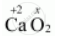CBSE (Science) Class 11CBSE
Share

# Assign Oxidation Numbers to the Underlined Elements in Each of the Following Species Cao2 - CBSE (Science) Class 11 - Chemistry

ConceptOxidation Number - Introduction

#### Question

Assign oxidation numbers to the underlined elements in each of the following species

CaO2

#### Solution 1

CaO2Then, we have

(+2) + 2(x) = 0

=> 2 + 2x = 0

=>x = -1

Hence, the oxidation number of O is – 1.

#### Solution 2

Let the oxidation number of CaO_2 be x

2 + 2x = 0    (∵ oxy No of a = +2)

x = -1

Thus oxidation number of O in CaO_2 = -1

Is there an error in this question or solution?

#### APPEARS IN

Solution Assign Oxidation Numbers to the Underlined Elements in Each of the Following Species Cao2 Concept: Oxidation Number - Introduction.
S